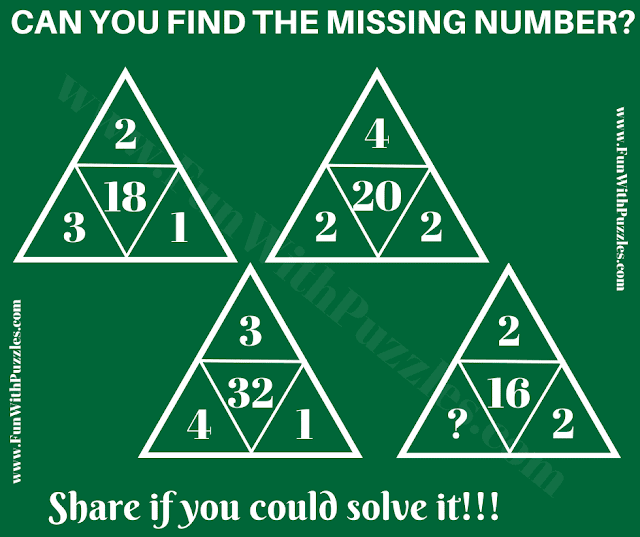This is a mind-challenging logic triangle puzzle question. In this puzzle question, there are 4 triangles. Each triangle contains four numbers. There is a hidden logical relationship between the four numbers in each triangle. Your challenge in this triangle puzzle question is to find the hidden logical reasoning. Can you decipher the logic and find the value of the missing number in this puzzle?
Please write down your answer to this puzzle in the comments along with the logical reasoning used to solve this puzzle. 'Meera V' is the first person to solve this puzzle correctly. Check out the comments to read the discussion on this puzzle.Can you find the value of the Missing Number?

The answer to this "Logic Triangle Puzzle Question", can be viewed by clicking on the answer button.

Unknown said...

4

Unknown said...

/4/

Rajesh Kumar said...

It will be great if you can also write down the logical reasoning used to solve this puzzle!

Meera V said...

2

My logic is as follows.
Central number = LHS Bottom number x (square of RHS bottom number + number at the top + LHS bottom number)

18 = 3 x (1x1 + 2 + 3 )
20 = 2 x ( 2x2 + 4 + 2)
32 = 4 x (1x1 + 3 + 4) and

16 = 2 x (2x2 + 2 + 2)

Rajesh Kumar said...

Thanks, Meera for your detailed comment.
Yes, your answer and logical reasoning to this triangle puzzle are correct. As you are the first person to correctly solve this puzzle, I have mentioned your name in this post.
Try to solve the other similar puzzles on this website. It will be nice to get your comments on these puzzles.